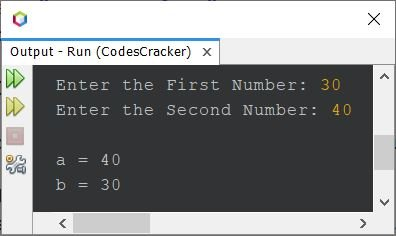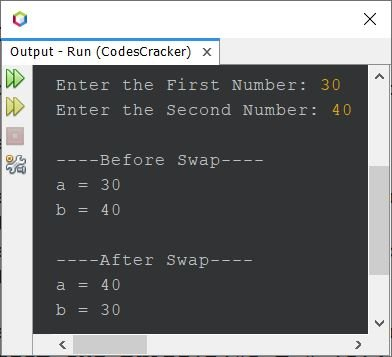# Java Program to Swap Two Numbers

This article covers a program in Java that swaps two numbers entered by user at run-time of the program.

## Swap Two Numbers using Third Variable in Java

The question is, write a Java program to swap any two given numbers. The number must be received by user at run-time. The program given below is its answer:

```import java.util.Scanner;

public class CodesCracker
{
public static void main(String[] args)
{
int a, b, temp;
Scanner s = new Scanner(System.in);

System.out.print("Enter the First Number: ");
a = s.nextInt();
System.out.print("Enter the Second Number: ");
b = s.nextInt();

temp = a;
a = b;
b = temp;

System.out.println("\na = " +a);
System.out.println("b = " +b);
}
}```

The snapshot given below shows the sample run of above Java program, on swapping of two given numbers, with user input 30 as first and 40 as second number:That is, the first number say 30 gets stored in a variable, and the second number say 40 gets stored in b variable. And using the statement:

`temp = a;`

Now temp holds the value of a, that is 30. Again using the following statement:

`a = b;`

The value of a becomes 40. And finally using the statement given below:

`b = temp;`

The value of temp, that is 30 gets initialized to b. So now, b holds the value of a, and a holds the value of b. That's it.

The above program can also be created in this way:

```import java.util.Scanner;

public class CodesCracker
{
public static void main(String[] args)
{
Scanner s = new Scanner(System.in);

System.out.print("Enter the First Number: ");
int a = s.nextInt();
System.out.print("Enter the Second Number: ");
int b = s.nextInt();

System.out.println("\n----Before Swap----");
System.out.println("a = " +a);
System.out.println("b = " +b);

int temp = a;
a = b;
b = temp;

System.out.println("\n----After Swap----");
System.out.println("a = " +a);
System.out.println("b = " +b);
}
}```

Here is its sample run with same user input as of previous program's sample run:## Swap Two Numbers without using Third Variable in Java

This program does not uses any third variable like temp to swap two numbers. Rather it uses the simple addition and subtraction operation to do the job.

```import java.util.Scanner;

public class CodesCracker
{
public static void main(String[] args)
{
Scanner s = new Scanner(System.in);

System.out.print("Enter the First Number: ");
int numOne = s.nextInt();
System.out.print("Enter the Second Number: ");
int numTwo = s.nextInt();

System.out.println("\n----Before Swap----");
System.out.println("numOne = " +numOne);
System.out.println("numTwo = " +numTwo);

numOne = numOne + numTwo;
numTwo = numOne - numTwo;
numOne = numOne - numTwo;

System.out.println("\n----After Swap----");
System.out.println("numOne = " +numOne);
System.out.println("numTwo = " +numTwo);
}
}```

## Swap Two Numbers using Function in Java

This program uses a user-defined function named swap() that takes two arguments. The first argument refers to the first number, whereas the second argument refers to the second number. The function swaps the two passed arguments and prints the values after swap.

```import java.util.Scanner;

public class CodesCracker
{
public static void main(String[] args)
{
Scanner s = new Scanner(System.in);

System.out.print("Enter the First Number: ");
int a = s.nextInt();
System.out.print("Enter the Second Number: ");
int b = s.nextInt();

System.out.println("\n----Before Swap----");
System.out.println("a = " +a);
System.out.println("b = " +b);

swap(a, b);
}

public static void swap(int x, int y)
{
int z;
z = x;
x = y;
y = z;

System.out.println("\n----After Swap----");
System.out.println("a = " +x);
System.out.println("b = " +y);
}
}```

## Swap Two Numbers using Bitwise Operator in Java

This program uses Bitwise operator to do the same job, that is, swapping of two numbers.

```import java.util.Scanner;

public class CodesCracker
{
public static void main(String[] args)
{
Scanner s = new Scanner(System.in);

System.out.print("Enter the First Number: ");
int a = s.nextInt();
System.out.print("Enter the Second Number: ");
int b = s.nextInt();

System.out.println("\n----Before Swap----");
System.out.println("a = " +a);
System.out.println("b = " +b);

a = a^b;
b = a^b;
a = a^b;

System.out.println("\n----After Swap----");
System.out.println("a = " +a);
System.out.println("b = " +b);
}
}```

Note - The ^ is a Bitwise XOR or Bitwise Exclusive OR operator. To learn about this operator, refer to Bitwise Operators.

#### Same Program in Other Languages

Java Online Test

« Previous Program Next Program »